• GHT（广义霍夫曼变换）

千次阅读 2018-11-02 16:03:03
研一的生活真的是精彩而又...借着上周工业视觉检测这门课，我认真的学习了一下霍夫曼变换与广义霍夫曼变换，想借着这个没课的下午做个简短记录。 1）首先，什么是霍夫曼变换？ 我的霍夫曼变换就是将空间坐标系里的...

研一的生活真的是精彩而又充实忙的跟狗一样，每天在学新的东西，忙的不知所措，还是决定要抽出空来，自己学习，好久都没有写博客了，我觉得这是自己的损失，无外乎两种情况：没有新的学习以及学习了却没有及时进行记录，两者都很可怕。

借着上周工业视觉检测这门课，我认真的学习了一下霍夫曼变换与广义霍夫曼变换，想借着这个没课的下午做个简短记录。

1）首先，什么是霍夫曼变换？

我的霍夫曼变换就是将空间坐标系里的图形转换到另外一个坐标系去表示。这么讲可能有的朋友会很迷茫，你在说什么？

换个说法，通常我们会用（x，y）来描述一个点的坐标，集中注意力了朋友，这个公式很关键

那么如果我们已知x，y，我们将和p视为变量，我们就可以发现这个结论，空间坐标系里面的一点，可以用参数坐标系里面的一条线来表示.而空间域的一条直线，通过变换发现成了参数域的一个点？是不是有懵逼了，别慌，我们来个example：

比如这条直线，当x，y都为1的时候，这个方程表示为：

作图的话就像下面这样：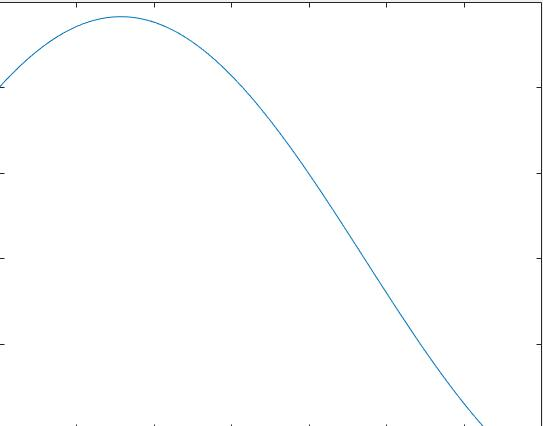然后我们继续添加点，可以看到图片变化成这样：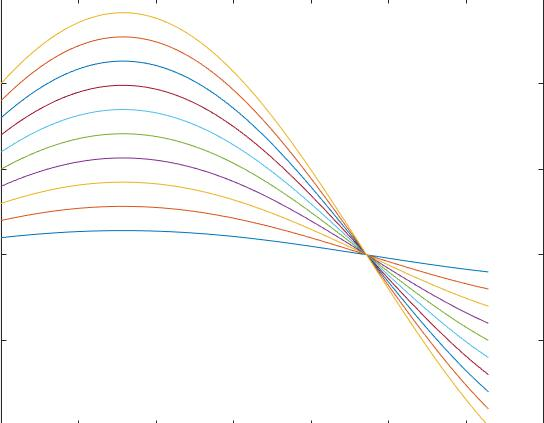我们可以发现最后所有点变成的直线都交于一点，那么是不是就可以这样推断，在空间域这些所有的点对应的是同一条直线，而转换到参数空间之后，这些所有的曲线交于的同一点就是空间域的那条直线。通过这种方法我们就可以进行直线检测了。

ojbk，当然不止直线检测，所有凡是可以用解析式表达出来的图形，都可以通过霍夫曼变换来检测。甚至现在都不需要我们自己写代码了opencv的库就可以了。

2）为什么要有广义霍夫曼变换（GFT）

当检测的几何形状为直线，椭圆，圆时，我们通过霍夫曼变换终究可以将他们在一个有限维的参数空间中表示出来。

但是当所要检测的形状为一些不规则图形，比如字母，或者是一只水杯，我们就很难用一个数学解析式去描述出这个形状。这时候霍夫曼变换就很难解决这个问题。

但是通过广义霍夫曼变换，这类的问题却得到了很好的解决。

3）如何进行GHT？

分为三部分：a）对不规则形状进行描述     b）对图像中的不规则图像进行检测

c）检测中如何实现算法对旋转，缩放变换的通用性

a)对于不规则形状的描述主要是如下图所示，将要检测的任意形状称为模板，在模板上选择一个中心点，对于模板边缘每个点计算切线与x轴夹角，称为角。然后利用这个角建立一个R-table。每个角都是R-table的一个索引。然后对这个边缘点计算他与参考点的长度以及连线与x轴的夹角，作为相应下的一个数据对存进去。通过这个R-table我们就获得了这个模板所有的位置信息。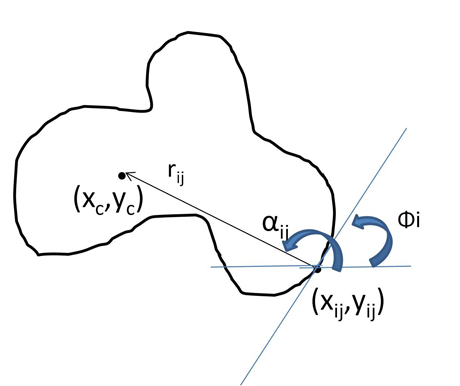b)但是如果待检测图像与模板存在角度与大小的差异，那么我们该怎么适应这种差异呢?

老规则上图、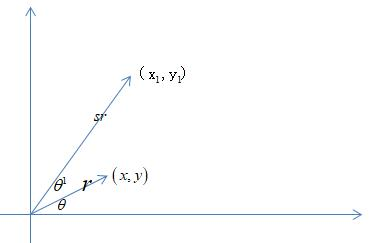(x,y)是为模板上的原点，而则是变换后图像上与原点匹配的一点。我们来探寻他们之间的关系：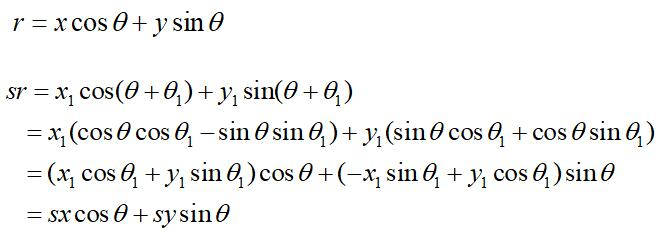那么如何使用s和来表示呢？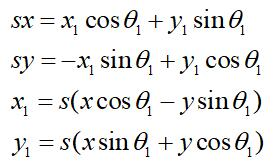c)最终的匹配过程可以描述如下：

1、将模板和待检测图像转化为灰度图

2利用sobel或者candy算子对模板和待检测图像进行边缘提取

3、对模板计算相对应的R-table

4、建立一个四维的累加器表（Accumulator table

5、对于待检测图像上的每个边缘点，计算对应的梯度方向。然后在R-table中检索相应的梯度值。

6、设定一个检测中旋转和缩放的范围。

7、对于该梯度值下所有的参数对，计算出相应的伪参考点坐标。

8假定我们要检测的对象都是经历过变换的，对于该坐标，对所有可能的旋转角度和缩放尺度，利用上页公式计算实际参考点位置。

9、对于累加器表中对应的累加器表的数值加一。这些都是如果该点属于要检测图像上的一点，可能的参考点位置。

10、对于累加器表中的点找局部最大值，如果大于阈值T，就认为该点作为中心，检测到一个待检测图像。

这是我做出来的结果展示：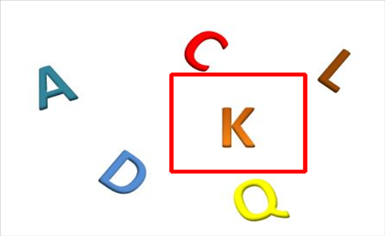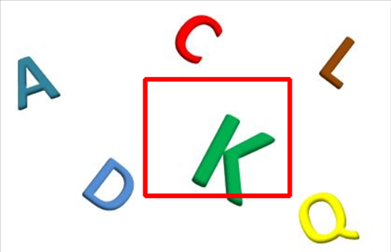4）人，还是要学会思考的，我觉得这个算法还有以下可以改进的地方：

1、广义霍夫曼变换中采用的累加表运用率很低，大部分的点都没有利用到却一直占据着内存空间

2、在广义霍夫曼变换中，论文中提出的方法只采用了一个点，但是在实验中有时情况比较复杂时还是会出现检测不到的状况，那么是不是可以考虑增加参考点的个数。

3、在程序中，通常是采用选取累加表中极大值的做法，这种方式通常只能检测出一个目标。如果把这个换为输出大于阈值的所有目标点，并依据局部极大值抑制或者检测框的重复率来确定最终目标的个数。

但是我真的是没空去做这些，等哪天有空了，我会去一一实现一下他们。

that‘s all。

我是钱多多，原创转载请征求本人意见，欢迎大家有空多交流。

学术交流可以关注我的公众号，后台留言，粉丝不多，看到必回。卑微小钱在线祈求展开全文• import cv2 import numpy as np img = cv2.imread("hd.jpeg", 0) img = cv2.GaussianBlur(img,(3,3),0) edges = cv2.Canny(img, 50, 150, apertureSize = 3) lines = cv2.HoughLines(edges,1,np.pi/10,118) #这...
import cv2
import numpy as np

img = cv2.GaussianBlur(img,(3,3),0)
edges = cv2.Canny(img, 50, 150, apertureSize = 3)
lines = cv2.HoughLines(edges,1,np.pi/10,118) #这里对最后一个参数使用了经验型的值
result = img.copy()

for m in range(lines.ndim):
for line in lines[m]:
rho = line #第一个元素是距离rho
theta= line #第二个元素是角度theta
#	print rho
#	print theta
if  (theta < (np.pi/4. )) or (theta > (3.*np.pi/4.0)): #垂直直线
#该直线与第一行的交点
pt1 = (int(rho/np.cos(theta)),0)
#该直线与最后一行的焦点
pt2 = (int((rho-result.shape*np.sin(theta))/np.cos(theta)),result.shape)
#绘制一条白线
cv2.line( result, pt1, pt2, (255))
else: #水平直线
# 该直线与第一列的交点
pt1 = (0,int(rho/np.sin(theta)))
#该直线与最后一列的交点
pt2 = (result.shape, int((rho-result.shape*np.cos(theta))/np.sin(theta)))
#绘制一条直线
cv2.line(result, pt1, pt2, (255), 1)

cv2.imshow('Canny', edges )
cv2.imshow('Result', result)
cv2.waitKey(0)
cv2.destroyAllWindows()展开全文• 该课题为基于Matlab霍夫曼变换的表盘读数识别。识别的意义在于我们可以假设，在一些危险的场景我们人员无法靠近，那么可以在仪表旁边安装一台摄像机，这个摄像机可以实时的监测仪表，当仪表上面的数值到达某一个数值...matlab
• 该课题为基于Matlab霍夫曼变换的表盘读数识别。识别的意义在于我们可以假设，在一些危险的场景我们人员无法靠近，那么可以在仪表旁边安装一台摄像机，这个摄像机可以实时的监测仪表，当仪表上面的数值到达某一个数值...matlab
• 基于MATLAB霍夫曼变换的答题卡识别方法一、背景随着科技的发展，电子与计算机技术的进步，答题卡的出现大大减轻教学工作者们批改试卷的工作量。答题卡是光标阅读机输入信息的载体，是配套光标阅读机的各种信息录入...

基于MATLAB霍夫曼变换的答题卡识别方法

一、背景

随着科技的发展，电子与计算机技术的进步，答题卡的出现大大减轻教学工作者们批改试卷的工作量。答题卡是光标阅读机输入信息的载体，是配套光标阅读机的各种信息录入表格的总称。 答题卡将用户需要的信息转化为可选择的选项，供用户涂写。OMR是用光学扫描的方法来识别按一定格式印刷或书写的标记，并将其转换为计算机能接受的电信号的设备，并根据信息点的涂与未涂和格式文件设置将信息还原。因此，如何将答题卡填涂的黑色区域识别出来并使用计算机进行处理是极为关键的。本论文探索了有效识别答题卡的方法，以matlab为工具，基于数字图像处理技术对答题卡填涂区域进行了识别，并对识别的结果进行了处理，得到了结果。本论文利用Hough变换的直线检测技术检测图像的倾斜度，判断图像是否倾斜，对存在倾斜的图像进行旋转校正。最终实现答题卡答案的定位和检测。论文使用像素统计方法进行识别，利用黑白颜色灰度值的巨大差异对二值图像的灰度值进行累加并进行阈值判定，识别错误效率极低，能够准确的识别答题卡的涂卡标记。

二、总体方案设计

说明系统运行环境，编程软件平台，编码算法原理，算法流程图设计

本系统运行在当今主流的Windows7系统，使用较新的MATLAB2014a进行设计。在进行旋转校正时，先使用Hough变换检测出答题卡边缘直线，调用lines函数的参数得到倾斜角度并进行旋转变换从而消除答题卡倾斜状态。将扫描部分分为7个扫描区域，分别使用像素检索技术，对各个检索区域内的灰度值进行累加，并对累加和进行阈值判定，从而得出检索区域颜色，完成判定。最后再与标准答案进行比对，得出成绩。

流程图：

开始

图像旋转

图像倾斜吗？

是 是

分别依次统计各大扫描区域内各个检测区域的灰度值

阈值判定，得出所检测区域颜色并赋值

与标准答案比对算出成绩

结束

三、程序设计

clc;clear;close;

imshow(A),title('原图');

gray=rgb2gray(A);

bw=edge(gray,'canny');%canny算子边缘检测得到二值边缘图像

[h,t,r]=hough(bw,'RhoResolution',0.5,'ThetaResolution',0.5); %Hough变换

xlabel('\theta'),ylabel('\rho');

axis on, axis normal,hold on;

P=houghpeaks(h,2);

x=t(P(:,2));y=r(P(:,1));

plot(x,y,'s','color','r'),title('');%获取并标出参数平面的峰值点

lines=houghlines(bw,t,r,P,'FillGap',5,'Minlength',7);%检测图像中的直线段

figure,imshow(gray);

hold on;

max_len=0;

for i=1:length(lines)

xy=[lines(i).point1;lines(i).point2];

plot(xy(:,1),xy(:,2),'LineWidth',2,'Color','g');%用绿色线段标注直线段

plot(xy(:,1),xy(1,2),'x','LineWidth',2,'Color','y');

plot(xy(:,1),xy(2,2),'x','LineWidth',2,'Color','r');%标注直线段端点

end

x=lines.theta;%由与图像边缘平行的直线段的斜率得到整个图像旋转的角度

B=imrotate(gray,x);%图像修正

figure,imshow(B),title('旋转后图像');

T=graythresh(B);%使用OTSU方法获得阀值T

result=im2bw(B,T);%二值化图像

figure,imshow(result),title('二值化后结果');

%扫描区域：在本程序中，有7个扫描区域，分别对应题号为1-5,6-10,11-15,16-20,21-25,26-30,31-35这几个区域

n=52;m=244;% 首先测得答题区域第一题A选项左上角的坐标（52,244）

s=60;t=90;%s为上下两个扫描区域的距离，t为左右两个扫描区域的距离。

P=4;Q=5;%P为字母项的个数（这里有ABCD共有4项），Q为每个小区域选项数。

interval_length=14;%左右相邻两题填涂区域左边线的距离

interval_width=9;%上下相邻两题填涂区域上边线的距离

length=11;%填涂区域的长度

width=6;%填涂区域的宽度

a2=zeros(4,5);%初始化灰度值统计矩阵

%第1-5题区域的检测

for P=1:4

for Q=1:5

%m=244;n=52;%第1-5题区域的检测的起始坐标（即第一题A选项左上角的坐标）

a1=result(m+(P-1)*(interval_width+1) :m+(P-1)*(interval_width+1)+width,n+(Q-1)*(interval_length+1):n+(Q-1)*(interval_length+1)+length);

%得到矩形区域内各像素的灰度值

sum1=sum(sum(a1));%计算统计的矩形区域内像素灰度值之和

a2(P,Q)=sum1;%多次循环后得到20个统计区域的灰度值，并依次放在a2矩阵中

end

end

a2(a2<80)=1;

a2(a2>=81)=0;%对灰度值统计矩阵里的数值进行处理，大于某一阀值的值赋1，其余的赋0。

%在此程序中，涂黑则相应数值为1。

result1_5=a2;%存储1-5题的结果

%第6-10题区域的检测

for P=1:4

for Q=1:5

a1=result(m+s*1+(P-1)*(interval_width+1) :m+s*1+(P-1)*(interval_width+1)+width,n+(Q-1)*(interval_length+1):n+(Q-1)*(interval_length+1)+length);

sum1=sum(sum(a1));

a2(P,Q)=sum1;

end

end

a2(a2<80)=1;

a2(a2>=81)=0;

result6_10=a2; %存储6-10题的结果

%第11-15题区域的检测

for P=1:4

for Q=1:5

a1=result(m+(P-1)*(interval_width+1) :m+(P-1)*(interval_width+1)+width,n+t*1+(Q-1)*(interval_length+1):n+t*1+(Q-1)*(interval_length+1)+length);

sum1=sum(sum(a1));

a2(P,Q)=sum1;

end

end

a2(a2<80)=1;

a2(a2>=81)=0;

result11_15=a2; %存储11-15题的结果

%第16-20题区域的检测

for P=1:4

for Q=1:5

a1=result(m+s*1+(P-1)*(interval_width+1) :m+s*1+(P-1)*(interval_width+1)+width,n+t*1+(Q-1)*(interval_length+1):n+t*1+(Q-1)*(interval_length+1)+length);

sum1=sum(sum(a1));

a2(P,Q)=sum1;

end

end

a2(a2<80)=1;

a2(a2>=81)=0;

result16_20=a2; %存储16-20题的结果

%第21-25题区域的检测

a2(a2<80)=1;

a2(a2>=81)=0;

result21_25=a2; %存储21-25题的结果

%第26-30题区域的检测

for P=1:4

for Q=1:5

a1=result(m+s*3+(P-1)*(interval_width+1) :m+s*3+(P-1)*(interval_width+1)+width,n+t*1+(Q-1)*(interval_length+1):n+t*1+(Q-1)*(interval_length+1)+length);

sum1=sum(sum(a1));

a2(P,Q)=sum1;

end

end

a2(a2<80)=1;

a2(a2>=81)=0;

result26_30=a2; %存储26-30题的结果

%第31-35题区域的检测

for P=1:4

for Q=1:5

a1=result(m+s*4+(P-1)*(interval_width+1) :m+s*4+(P-1)*(interval_width+1)+width,n+t*1+(Q-1)*(interval_length+1):n+t*1+(Q-1)*(interval_length+1)+length);

sum1=sum(sum(a1));

a2(P,Q)=sum1;

end

end

a2(a2<80)=1;

a2(a2>=81)=0;

result31_35=a2; %存储31-35题的结果

%附加一个统计错误的并显示分数的部分，假定正确答案是35个C

T=zeros(4,35);%初始化矩阵

T(1,:)=1;%设定正确答案的参数

wrongx=sum(mark1~=0);%当结果选错时，每一列将分别多一个1与-1。选择正确时，此列都是0。

A=sum(sum(wrongx));%统计非零的结果。

wrong=A/2;%由于-1与1是成对出现的，所以需要将上一步所得结果除以2.

score=35-wrong;%算出结果

fprintf('选择题得分为%d',score);%在command窗口输出成绩

四、模块测试与分析

1、首先进行Hough变换，得到Hough变换矩阵如下所述（见图1）。图1

可以观察到2个峰值。

2、使用Houghlines函数检测直线，得到如下结果（见图2）图2

并使用lines.theta语句得到直线角度，即为图像倾斜角度。

x=lines.theta x =4

3、修正图像（如图3所示）图3

这时观察到倾斜的图像已经得到改正。

4、在二值化后使用像素检索技术对灰度值进行统计。分为7个扫描区域，如图4中7个方框所示。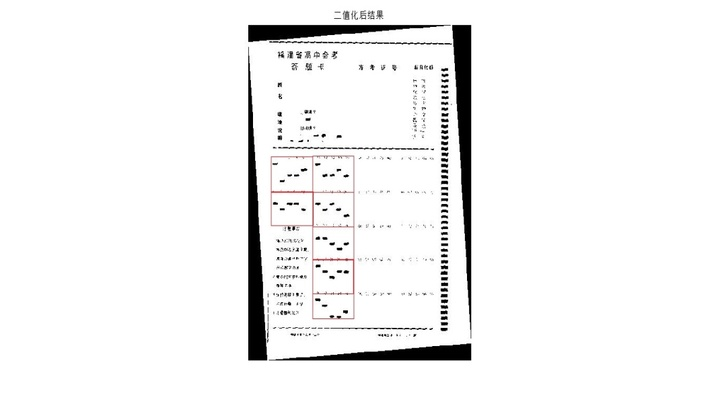图4

5、进行灰度统计，得到结果矩阵。最后处理由fprintf语句得到分数。

展开全文算法 计算机视觉 opencv matlab python
• 课题为matlab答题卡识别，带界面GUI框架。需要您具备一定基础。可以识别学号，学科，填涂区的ABCD等，跟标准分数对比，判断及格与否。带界面GUI框架。需要您具备一定基础。请多包涵。matlab
• 基于matlab的答题卡识别，算法：霍夫曼。步骤比较多：灰度化，边缘检测，二值化，霍夫曼变换，图像校准，分割，识别。带界面GUI，识别单选，多选，学号等。可以跟标准答案对比。
• 该课题为基于霍夫曼变换的仪表示数识别。平台是matlab。可以框定直线，计算仪表的示数，再由人机交互界面。matlab
• //霍夫曼直线检测 Point pt1, pt2; HoughLines(src_gray, lines, 1, CV_PI / 180, 150, 0, 0); for (size_t i = 0; i (); i++) { // 极坐标中的r长度 float rho = lines[i]; // 极坐标中的角度 ...

前言

1.直线检测在好多实现应用中能用到到，我之前做过扫描件检测时用直线检测来处理判断页面是否水平，还有在辅助驾驶中的车道偏离预警系统也有过应用。
2.我的编程环境是Windows 7 64位，IDE是VS2015，配置了OpenCV3.3与OpenCV_Contrib,实现语言是C++。是于如果配置以上的环境，可以看我之前写的博文。

一、概述

1.直线检测的原理介绍
（1）对于直角坐标系中的任意一点A(x0,y0)，经过点A的直线满足Y0=kX0+b.(k是斜率，b是截距)。
（2）那么在X-Y平面过点A(x0,y0)的直线簇可以用Y0=k
X0+b表示，但对于垂直于X轴的直线斜率是无穷大的则无法表示。因此将直角坐标系转换到极坐标系就能解决该特殊情况。
（3）在极坐标系中表示直线的方程为ρ=xCosθ+ySinθ（ρ为原点到直线的距离）。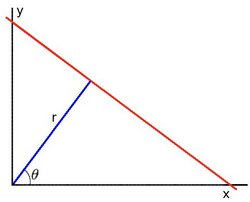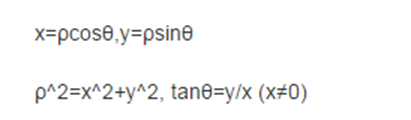（4）对于任意一条直线上的所有点来说，变换到极坐标中，从[0~360]空间，可以得到r的大小，属于同一条直线上点在极坐标空(r, theta)必然在一个点上有最强的信号出现，根据此反算到平面坐标中就可以得到直线上各点的像素坐标。从而得到直线。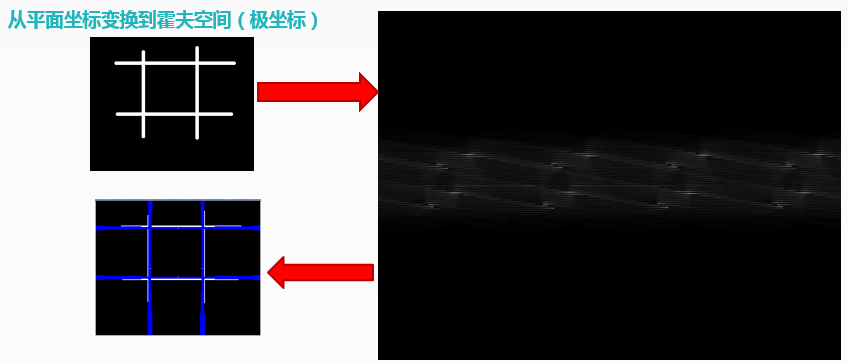2.OpenCV封装有直线检测的函数，HoughLines（）和HoughLinesP（），它们都能实现直线检测，差别是：HoughLines（）函数使用标准的Hough变换HoughLinesP（）函数使用概率Hough变换，即只通过分析点的子集并估计这些点都属于一条直线的概率，这在计算速度上更快。
（1）HoughLines（）函数说明：
HoughLines(
InputArray src, // 输入图像，必须8-bit的灰度图像
OutputArray lines, // 输出的极坐标来表示直线
double rho, // 生成极坐标时候的像素扫描步长
double theta, //生成极坐标时候的角度步长，一般取值CV_PI/180
int threshold, // 阈值，只有获得足够交点的极坐标点才被看成是直线
double srn = 0;// 是否应用多尺度的霍夫变换，如果不是设置0表示经典霍夫变换
double stn = 0;//是否应用多尺度的霍夫变换，如果不是设置0表示经典霍夫变换
double min_theta = 0; // 表示角度扫描范围 0 ~180之间， 默认即可
double max_theta = CV_PI
)
（2）HoughLinesP（）函数说明：
HoughLinesP(
InputArray src, // 输入图像，必须8-bit的灰度图像
OutputArray lines, // 输出的极坐标来表示直线
double rho, // 生成极坐标时候的像素扫描步长
double theta, //生成极坐标时候的角度步长，一般取值CV_PI/180
int threshold, // 阈值，只有获得足够交点的极坐标点才被看成是直线
double minLineLength = 0;// 最小直线长度
double maxLineGap = 0;// 最大间隔
)

二、代码演示

1.传入一张图像，把所有直线画出来。

void lineDetection(string image_path)
{
Mat src, src_gray, dst;
if (!src.data)
{
std::cerr << "无法打开图像文件！" << endl;
return;
}

namedWindow("input image", CV_WINDOW_AUTOSIZE);
namedWindow("hough-line-detection", CV_WINDOW_AUTOSIZE);
imshow("input image", src);

//边缘检测
Canny(src, src_gray, 150, 200);
//灰度化
cvtColor(src_gray, dst, CV_GRAY2BGR);

vector<Vec2f> lines;
//霍夫曼直线检测
Point pt1, pt2;
HoughLines(src_gray, lines, 1, CV_PI / 180, 150, 0, 0);
for (size_t i = 0; i < lines.size(); i++)
{
// 极坐标中的r长度
float rho = lines[i];
// 极坐标中的角度
float theta = lines[i];
double a = cos(theta), b = sin(theta);
double x0 = a*rho, y0 = b*rho;
// 转换为平面坐标的四个点
pt1.x = cvRound(x0 + 1000 * (-b));
pt1.y = cvRound(y0 + 1000 * (a));
pt2.x = cvRound(x0 - 1000 * (-b));
pt2.y = cvRound(y0 - 1000 * (a));
line(dst, pt1, pt2, Scalar(0, 0, 255), 1, CV_AA);
}

imshow("matt", src);

imshow("hough-line-detection", dst);

waitKey(0);
}

2.运行结果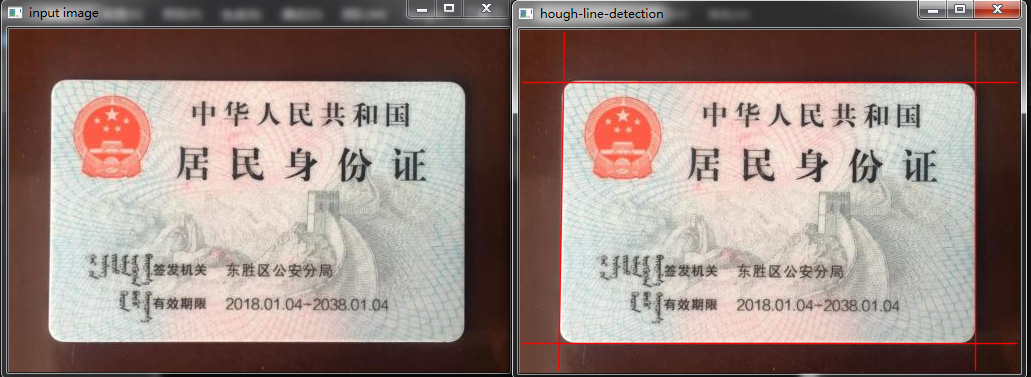结语

1.例子很简单，没有什么难度，但应用时肯定要按自己的数据去做相关参数的设定。
2.关于工程的源码，运行程序时的bug,都可以加这个群(487350510)互相讨论学习。

展开全文霍夫直线检测 OpenCV3 Houghline 计算机视觉
• 该课题为基于霍夫曼变换的仪表示数识别。平台是matlab。可以框定直线，计算仪表的示数，再由人机交互界面。matlab
• 该个体为基于MATLAB的答题卡识别。可以识别学号填读区域学科以及跟标准答案进行对比，带有人际交互界面。可在人际交互界面的基础之上进行相应的拓展。matlab
• 该课题为基于霍夫曼变换的仪表示数识别。平台是matlab。可以框定直线，计算仪表的示数，再由人机交互界面。matlab
• 课题为基于Matlab的仪表指数识别系统。通过霍夫曼变换的方法。可以去框定直线计算角度根据量程的比例换算得到计数。matlab
• 这个课题为基于MATLAB的答题卡识别，采用hough变换原理，定位答题卡的ABCD字符，然后识别。得到结果和excel标准分数进行对比，可以得出得分，判断及格与否，也可以查询某个考生号进行分数查询。带界面GUI框架。需要...matlab
• matlab霍夫曼算法的答题卡识别，识别单选，多选，学号，科目，分数。带界面
• Hough变换是经典的检测直线的算法。其最初用来检测图像中的直线，同时也可以将其扩展，以用来检测图像中简单的结构。 import cv2 import numpy as np img = cv2.imread("road.jpg", 0) img = cv2.GaussianBlur(img...
• matlab仪表示数检测，表盘刻度识别。通过霍夫曼变换方法，检测定位指针位置，检测角度，利用相应的尺度比例换算为示数。带GUI界面。
• 这个课题为基于MATLAB的答题卡识别，采用hough变换原理，定位答题卡的ABCD字符，然后识别。得到结果和excel标准分数进行对比，可以得出得分，判断及格与否，也可以查询某个考生号进行分数查询。带界面GUI框架。需要...matlab
• MATLAB霍夫曼变换答题卡应用（GUI）.zip
• MATLAB霍夫曼变换表盘读书识别[GUI].zip
• 该个体为基于MATLAB的答题卡识别。可以识别学号填读区域学科以及跟标准答案进行对比，带有人际交互界面。可在人际交互界面的基础之上进行相应的拓展。matlab
• MATLAB霍夫曼变换的答题卡识别系统，带界面GUI，检测答题卡的学号，科目，单选多选，最后跟标准答案相比，对比是否及格。
• MATLAB 霍夫曼变换答题卡识别（GUI，万字文稿）.zip
• 基于MATLAB霍夫曼变换的车道线检测系统

千次阅读 热门讨论 2020-11-30 15:56:28
对于直车道线，利用Hough变换进行直线的提取，筛选并绘制出离车辆最近的两条直车道线；而对于弯曲车道线，则通过最小二乘法利用抛物线模型进行曲线拟合，并根据抛物线二次项系数判别弯道转向。...算法 计算机视觉 机器学习 python opencv...